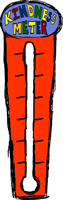[private_Basic]
What Have You Done That Smells Great?
[/private_Basic]
user_email),”=”));

/* Here, we check to see if this user is in the legacy table that ties in with the graph. If not, we add them to the table with basic info.
There are better ways to handle this, but this way will work for now. */
/* Begin adding user to legacy users table. */
if (!empty(\$current_user->user_email)) {
putenv(‘TZ=US/Eastern’); // Set the timezone.

\$query=”SELECT id FROM ids_users WHERE email = ‘\$current_user->user_email'”;
\$result=mysql_query(\$query) or die(mysql_error());

if (mysql_num_rows(\$result) == 0) {
\$query = “INSERT INTO ids_users (first_name, last_name, email, active, reg_date)
VALUES (‘\$current_user->user_firstname’, ‘\$current_user->user_lastname’, ‘\$current_user->user_email’, 1, ‘\$today’)”;
\$result = mysql_query(\$query) or die(mysql_error());
}
}
/* End adding user to legacy users table. */

/* Get the totals for the graph. */
\$query=”SELECT sum(count) as count_total, (SELECT sum(count) FROM nice_acts_counter, ids_users WHERE user_id = ids_users.id AND email = ‘\$current_user->user_email’) as count FROM nice_acts_counter”;
\$result_total=mysql_query(\$query) or die(mysql_error());
\$row_total = mysql_fetch_array(\$result_total, MYSQL_ASSOC);
\$user_total = \$row_total[‘count’] * 3;
\$all_total = round(\$row_total[‘count_total’] / 33.00); /* This setting should make the counter reset at 5,000. (make it 33.33 for 5,000 and 62 for 10 k) */

echo “\n\n”;

\$max = 150; /* This is the height of the inside of the meter. */
/* Count how many times the user has filled the meter and reset the meter. */
if (\$user_total > \$max) {
\$u_times = floor(\$user_total / \$max);
\$um_total = \$user_total – (\$u_times * \$max);
} else {
\$um_total = \$user_total;
}

/* Do the same resetting for the everyone meter count. */
if (\$all_total > \$max) {
\$a_times = floor(\$all_total / \$max);
\$am_total = \$all_total – (\$a_times * \$max);
} else {
\$am_total = \$all_total;
}

\n”;
// echo ‘User email: ‘ . \$current_user->user_email . “
\n”;
?>

display_name)) {

echo “Hi \$current_user->display_name!”;
if (isset(\$_GET[‘cs’])) {
?>

Log Out

\n”;
} ?>0) { ?>

“; */ ?>
Filled“; ?>
Filled

Everyone’s Total:Fresh-Smelling Act Yes Your Total Everyone’s Total

“Everyday Kindness”, “EK” => “Environmental Kindness”, “KO” => “Kindness at the Office/School”, “CK” => “Community Kindness”, “KR” => “Kindness On the Road”, “FK” => “Family Kindness”, “F” => “Friends”, “SK” => “Self Kindness”);
// foreach (\$cats as \$key => \$val) {
\$query=”SELECT id, act FROM nice_acts WHERE cat = ‘\$cat’ ORDER by act”;
\$result=mysql_query(\$query) or die(mysql_error());

echo ”

\$cats[\$cat]

\n”;

while (\$row = mysql_fetch_assoc(\$result)) {
\$al .= “\$row[id],”;
\$query=”SELECT sum(count) as count_total, (SELECT count FROM nice_acts_counter, ids_users WHERE user_id = ids_users.id AND email = ‘\$current_user->user_email’ AND nice_acts_id = \$row[id]) as count FROM nice_acts_counter WHERE nice_acts_id = \$row[id]”;

\$result_c=mysql_query(\$query) or die(mysql_error());
\$row_c = mysql_fetch_array(\$result_c, MYSQL_ASSOC);

if (\$r_num == 2) \$r_num = 1; else \$r_num = 2;
?>

display_name))
?>